# Create lines from points and cut rectangle with line and assign attributes

1639
4
03-28-2017 01:15 PMNew Contributor

Ok, attached I have the point data and the rectangle as polygon. I want to draw vertical line from each point (from the top to the bottom) and keep the information written in the LN column. After I want to make single polygons (resulting from the underlying rectangle and the lines together) and also keep the LN information in the single polygons. So in the end it shoudl be 13 single polygons, I hope you understand what I mean.

Best,

Tim

1 Solution

Accepted SolutionsbyEsri Esteemed Contributor

Hi Tim Gattinger , see below the code I created based on the information in the point shapefile:

#-------------------------------------------------------------------------------
# Name:        create_rectangles.py
# Purpose:
#
# Author:      Xander
#
# Created:     01-04-2017
#-------------------------------------------------------------------------------
import arcpy
import os

def main():
arcpy.env.overwriteOutput = True

# parameters
fc_in = r'C:\GeoNet\GW1\ref_kal_ln\ref_kal_ln.shp'
fc_out = r'C:\GeoNet\GW1\rectangles_v01.shp'
where = '"ID" <14'
fld_x_max = 'X'
fld_y_max = 'Y'
fld_width = 'width'
fld_height = 'length'

# create output featureclass
sr = arcpy.Describe(fc_in).spatialReference
CreateFeatureClassTemplate(fc_out, fc_in, "POLYGON", fc_in, sr)

# nested insert and search cursor
flds = GetAllFieldsWithoutSystemFields(fc_in)
with arcpy.da.InsertCursor(fc_out, flds) as curs_out:
with arcpy.da.SearchCursor(fc_in, flds, where) as curs:
for row in curs:
x_max = row[flds.index(fld_x_max)]
y_max = row[flds.index(fld_y_max)]
width = row[flds.index(fld_width)]
height = row[flds.index(fld_height)]

polygon = CreateRectangle(x_max, y_max, width, height, sr)
if polygon is not None:
lst_row = list(row)
lst_row = polygon
row_out = tuple(lst_row)
curs_out.insertRow(row_out)

def GetAllFieldsWithoutSystemFields(fc):
flds = [fld.name for fld in arcpy.ListFields(fc)]
desc = arcpy.Describe(fc)
fld_shape = desc.shapeFieldName
fld_oid = desc.OIDFieldName
for fld_name in [fld_shape, fld_oid]:
if fld_name in flds:
flds.pop(flds.index(fld_name))
flds.insert(0, 'SHAPE@')
return flds

def CreateFeatureClassTemplate(fc_out, fc_in, geomtype, template,  sr):
ws_name, fc_name = os.path.split(fc_out)
arcpy.CreateFeatureclass_management(ws_name, fc_name, geomtype, template, spatial_reference=sr)

def CreateRectangle(x_max, y_max, width, height, sr):
pnt1 = arcpy.Point(x_max - width, y_max - height)
pnt2 = arcpy.Point(x_max - width, y_max)
pnt3 = arcpy.Point(x_max, y_max)
pnt4 = arcpy.Point(x_max, y_max - height)
lst_pnts = [pnt1, pnt2, pnt3, pnt4, pnt1]
return arcpy.Polygon(arcpy.Array(lst_pnts), sr)

if __name__ == '__main__':
main()‍‍‍‍‍‍‍‍‍‍‍‍‍‍‍‍‍‍‍‍‍‍‍‍‍‍‍‍‍‍‍‍‍‍‍‍‍‍‍‍‍‍‍‍‍‍‍‍‍‍‍‍‍‍‍‍‍‍‍‍‍‍‍‍‍‍‍‍‍‍‍‍‍‍‍‍‍‍‍‍‍‍‍‍‍‍‍‍‍‍‍‍‍‍‍‍‍‍‍‍‍‍‍‍‍‍‍‍‍‍‍‍‍‍‍‍‍‍‍‍‍‍‍‍‍‍‍‍‍‍‍‍‍‍‍‍‍‍‍‍‍‍‍‍‍‍

The upper part of the shapefile looks like this: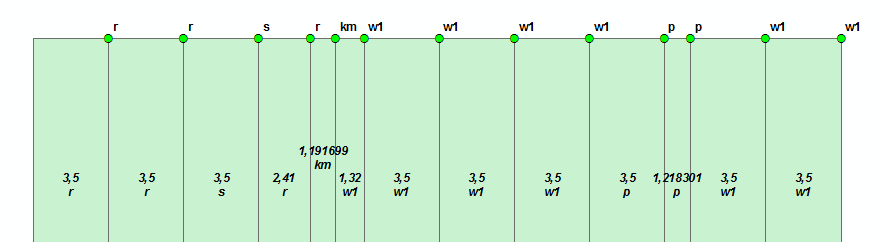The attribute table of the resulting shapefile (attributes are transferred):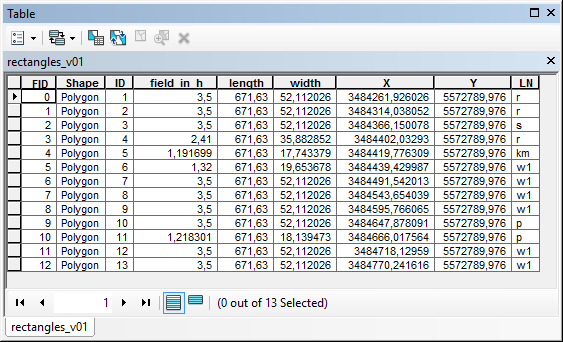I have attached the resulting shapefile for you to examine.

4 RepliesbyEsri Esteemed Contributor

When I look at the data I see that you are using a format (point featureclass) that is different from the original format used in the thread Create a line from points using attributes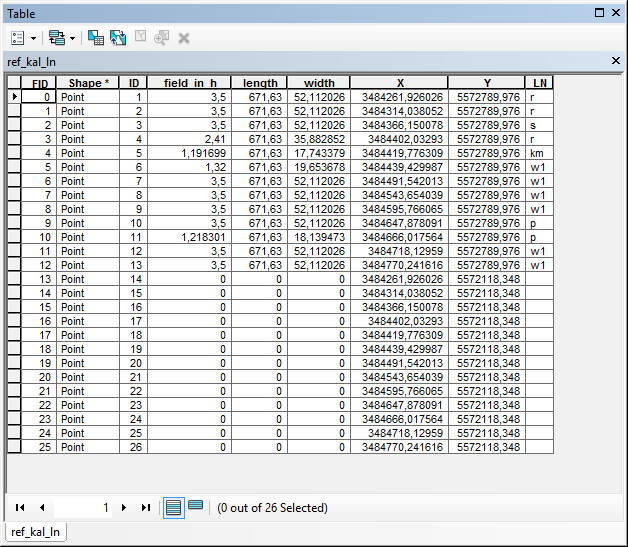I understand that you want to create vertical lines and use these to cut a rectangle in smaller parts in order to generate the smaller rectangles and assign values from the attributes of the input points. This may not be as straight forward as you hope, since the input points do not hold details on which points should be used together to form the line (although this will probably be those with the same X-coordinates):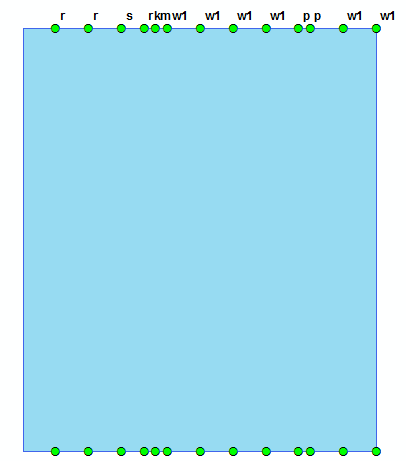I would like to suggest another input format (if possible) to make this process a lot easier. You basically have a constant Y (min and max) and a Xmin. When you know the width of each rectangle and you start with Xmin and apply the width you will be able to form the rectangles with little effort and it will be easy to maintain the attributes (for instance field LN):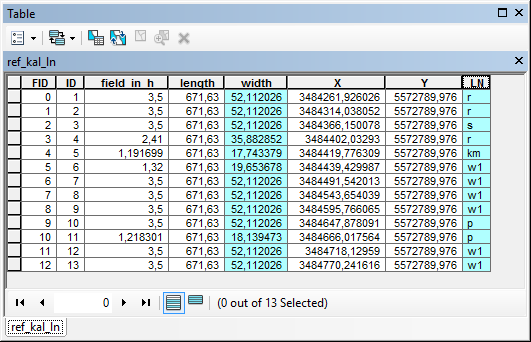Could you elaborate a little on what the data represents and where it comes from and what you want to achieve (apart from the smaller rectangles)?

Kind regards, XanderbyEsri Esteemed Contributor

Hi Tim Gattinger , see below the code I created based on the information in the point shapefile:

#-------------------------------------------------------------------------------
# Name:        create_rectangles.py
# Purpose:
#
# Author:      Xander
#
# Created:     01-04-2017
#-------------------------------------------------------------------------------
import arcpy
import os

def main():
arcpy.env.overwriteOutput = True

# parameters
fc_in = r'C:\GeoNet\GW1\ref_kal_ln\ref_kal_ln.shp'
fc_out = r'C:\GeoNet\GW1\rectangles_v01.shp'
where = '"ID" <14'
fld_x_max = 'X'
fld_y_max = 'Y'
fld_width = 'width'
fld_height = 'length'

# create output featureclass
sr = arcpy.Describe(fc_in).spatialReference
CreateFeatureClassTemplate(fc_out, fc_in, "POLYGON", fc_in, sr)

# nested insert and search cursor
flds = GetAllFieldsWithoutSystemFields(fc_in)
with arcpy.da.InsertCursor(fc_out, flds) as curs_out:
with arcpy.da.SearchCursor(fc_in, flds, where) as curs:
for row in curs:
x_max = row[flds.index(fld_x_max)]
y_max = row[flds.index(fld_y_max)]
width = row[flds.index(fld_width)]
height = row[flds.index(fld_height)]

polygon = CreateRectangle(x_max, y_max, width, height, sr)
if polygon is not None:
lst_row = list(row)
lst_row = polygon
row_out = tuple(lst_row)
curs_out.insertRow(row_out)

def GetAllFieldsWithoutSystemFields(fc):
flds = [fld.name for fld in arcpy.ListFields(fc)]
desc = arcpy.Describe(fc)
fld_shape = desc.shapeFieldName
fld_oid = desc.OIDFieldName
for fld_name in [fld_shape, fld_oid]:
if fld_name in flds:
flds.pop(flds.index(fld_name))
flds.insert(0, 'SHAPE@')
return flds

def CreateFeatureClassTemplate(fc_out, fc_in, geomtype, template,  sr):
ws_name, fc_name = os.path.split(fc_out)
arcpy.CreateFeatureclass_management(ws_name, fc_name, geomtype, template, spatial_reference=sr)

def CreateRectangle(x_max, y_max, width, height, sr):
pnt1 = arcpy.Point(x_max - width, y_max - height)
pnt2 = arcpy.Point(x_max - width, y_max)
pnt3 = arcpy.Point(x_max, y_max)
pnt4 = arcpy.Point(x_max, y_max - height)
lst_pnts = [pnt1, pnt2, pnt3, pnt4, pnt1]
return arcpy.Polygon(arcpy.Array(lst_pnts), sr)

if __name__ == '__main__':
main()‍‍‍‍‍‍‍‍‍‍‍‍‍‍‍‍‍‍‍‍‍‍‍‍‍‍‍‍‍‍‍‍‍‍‍‍‍‍‍‍‍‍‍‍‍‍‍‍‍‍‍‍‍‍‍‍‍‍‍‍‍‍‍‍‍‍‍‍‍‍‍‍‍‍‍‍‍‍‍‍‍‍‍‍‍‍‍‍‍‍‍‍‍‍‍‍‍‍‍‍‍‍‍‍‍‍‍‍‍‍‍‍‍‍‍‍‍‍‍‍‍‍‍‍‍‍‍‍‍‍‍‍‍‍‍‍‍‍‍‍‍‍‍‍‍‍

The upper part of the shapefile looks like this:The attribute table of the resulting shapefile (attributes are transferred):I have attached the resulting shapefile for you to examine.New Contributor

Dear Xander,

may I send you a message? Afaik in this community it only works if you would follow me..byEsri Esteemed Contributor